The COPULA Procedure

Hierarchical Archimedean Copula (HAC)

(Experimental)
Subsections:

Adopting the notations of Savu and Trede (2010), let
denote the total level of hierarchies and let
denote the dimension of the HAC. There are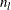distinct copulas at each level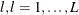. These copulas are indexed by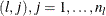. At each level, there are also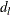variables,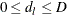and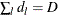. In the first step, all the variables at the lowest level are grouped into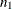subsets, each subset being an ordinary multivariate Archimedean copula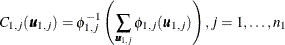where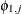is the generator of copula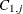,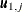denotes the variables that belong to copula, and the sum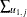is the sum over each variable in the subset. The copulascan be different Archimedean copulas for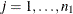. Then at the second level, the copulasthat are derived in the first level are aggregated as if they are individual variables. Suppose there are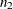copulas and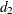variables,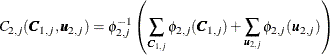where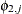denotes the generator of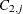and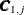represents the subset of copulas in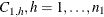, that is aggregated for copulafor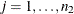. This structure continues until at level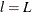a single copula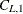aggregates all the copulas at its previous level,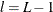.

A four-dimensional example that has total levels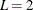and a structure shown in Figure 10.5 is defined as follows: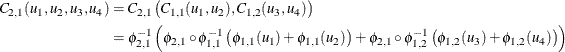Figure 10.5: Example Four-Dimensional Hierarchical Structure with Two Levels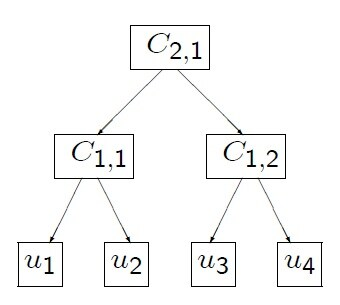Theorem 4.4 of McNeil (2008) states that the sufficient condition for a general hierarchical Archimedean structure to be a proper copula is that all appearing nodes of the form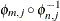have completely monotone derivatives. This condition places certain constraints on the copula parameters. In particular, if all the copulas in a hierarchical structure come from the Frank, Clayton, or Gumbel family, then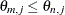for all j when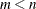. Intuitively, this means that rank correlation must be increasing as you move down the hierarchical structure.

The hierarchical Archimedean copulas available in the COPULA procedure are the hierarchical versions of the Clayton, Frank, and Gumbel copulas.

Simulation

A slightly modified version of the recursive algorithm from McNeil (2008) works for all valid hierarchical structures that have Clayton, Frank, or Gumbel generators:

1. Start at, and generate a random variable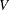with the distribution function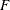with Laplace transform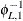.

2. For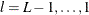, generate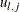from its parent hierarchy. For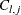, recursively call this algorithm with the proper inner generators that correspond to the copula family.

3. Return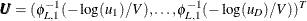.

Let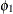be the outer generator and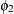the nested generator, and let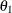and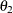be the respective generator parameters. Let v be a draw from distribution functionwith Laplace transform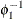. The inner copula generators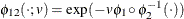and their corresponding Laplace transform distributions for the Clayton, Frank, and Gumbel family are summarized in Table 10.3.

Table 10.3: Inner Generators and Corresponding Distributions

Copula Type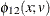Distribution with LT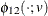Clayton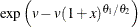Tiled stable

Gumbel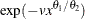Stable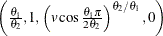Frank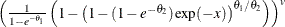No closed form

Note that when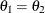, the inner generators for the Clayton and Gumbel family both simplify to the generator of the independence copula,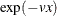. For more information about simulating from the distribution with the Laplace transform given by the inner generator for the Frank family, see Hofert (2011). For more information about how to simulate from a tilted stable distribution, see McNeil (2008).